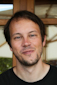# AIC(fit1)

4 posts / 0 newOffline
Joined: 07/31/2009 - 14:25
AIC(fit1)

would it be worth getting this to work for Mx as an accessor function for AIC, with the benefit of being consilient with other functions in R?
AIC(fit1)
Error in UseMethod("logLik") :
no applicable method for 'logLik' applied to an object of class "MxModel"Offline
Joined: 02/04/2010 - 20:45
AIC(...) and logLik(...)

I very much like the idea to place the class MxModel into the generic R framework for likelihood-based calculations. I hacked together the function and this seems to work ad hoc.

logLik.MxModel<-function(model)
{
ll <- NA
if (!is.null(model@output) & !is.null(model@output$Minus2LogLikelihood)) ll <- -0.5*model@output$Minus2LogLikelihood

if (!is.null(model@data))
attr(ll,"nobs") <- model@data@numObs
else
attr(ll,"nobs") <- NA
if (!is.null(model@output))
attr(ll,"df")<- length(model@output\$estimate)
else
attr(ll,"df") <- NA
class(ll) <- "logLik"
return(ll);
}

This can be used to obtain log-likelihood and/or AIC, e.g., by typing

model <- mxRun(mxModel(...))

logLik(model)
AIC(model)

There is one more thing. As far as I understand, R defines the AIC based on the -2loglikelihood and degrees of freedom equal to the freely estimated parameters in the model, whereas OpenMx defines AIC based on the chi^2 and the degrees of freedom equal to the difference of parameters between the model and the saturated model. The AIC difference between any two models will come out the same, either way, but this has great potential to confuse users. So, if this function was integrated into OpenMx, the different ways to calculate AIC should be stated in the summary()-function.Offline
Joined: 02/04/2010 - 20:45
Patch to OpenMx

I attach a patch to OpenMx (rev. 2295) that adds the capability as described above. With this patch applied (in trunk directory) and with adding the attached MxLogLik.R into subdirectory "R", I am able to obtain log-likelihood, AIC, and BIC via the generic accessors from the stats-package, e.g.:

model <- mxRun(mxModel(...))

logLik(model)
AIC(model)
BIC(model)Offline
Joined: 07/31/2009 - 14:25
great idea: let's roll this patch into the upcoming release!

It's much nicer for people if the standard over-loaded functions work.

Definately nice to be able to say AIC(model)

probably we should also implement a version of plot() which plots a model as a RAM graph...